# 44 Results

View
Selected filters:
• Equations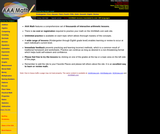Rating

-AAA Math features a comprehensive set of thousands of interactive arithmetic lessons.

-There is no cost or registration required to practice your math on the AAAMath.com web site.

-Unlimited practice is available on each topic which allows thorough mastery of the concepts.

-A wide range of lessons (Kindergarten through Eighth grade level) enables learning or review to occur at each individual's current level.

-Immediate feedback prevents practicing and learning incorrect methods, which is a common result of traditional homework and worksheets. Practice can continue as long as desired in a non-threatening format which helps build self-esteem and confidence.

-Please feel free to try the lessons by clicking on one of the grades at the top or a topic area on the left side of the page.

-Remember to add the site to your Favorite Places and please tell others about the site. It is an excellent way to learn or review math.

Subject:
Math
Material Type:
Activity/Lab
03/25/2019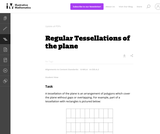Unrestricted Use
CC BY
Rating

This task examines the ways in which the plane can be covered by regular polygons in a very strict arrangement called a regular tessellation. These tessellations are studied here using algebra, which enters the picture via the formula for the measure of the interior angles of a regular polygon (which should therefore be introduced or reviewed before beginning the task). The goal of the task is to use algebra in order to understand which tessellations of the plane with regular polygons are possible.

Subject:
Math
Material Type:
Activity/Lab
Provider:
Illustrative Mathematics
Provider Set:
Illustrative Mathematics
Author:
Illustrative Mathematics
01/21/2013Unrestricted Use
CC BY
Rating

This task provides a simple but interesting and realistic context in which students are led to set up a rational equation (and a rational inequality) in one variable, and then solve that equation/inequality for an unknown variable.

Subject:
Math
Material Type:
Activity/Lab
Provider:
Illustrative Mathematics
Provider Set:
Illustrative Mathematics
Author:
Illustrative Mathematics
12/15/2012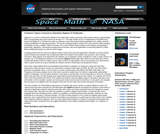Rating

This downloadable text features over 200 math problems that very closely follow the standard curriculum for high school Algebra 2 courses, but with a strong emphasis on space science and astronomy. Fourteen chapters featuring on-grade-level Algebra 2 concepts and skill areas including statistics, probability, conics, trigonometry, complex numbers and matrix algebra. Science topics are drawn from all areas of planetary, solar and astrophysics, in addition to space exploration and rocketry.

Subject:
Math
Physical Science
Material Type:
Lesson
Provider:
NASA
Provider Set:
Space Math
01/18/2011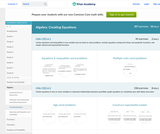Conditional Remix & Share Permitted
CC BY-NC-SA
Rating

This site teaches High Schoolers how to create equations through a series of 298 questions and interactive activities aligned to 5 Common Core mathematics skills.

Subject:
Math
Material Type:
Activity/Lab
Provider:
Provider Set:
10/10/2018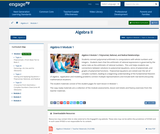Conditional Remix & Share Permitted
CC BY-NC-SA
Rating

Students connect polynomial arithmetic to computations with whole numbers and integers.  Students learn that the arithmetic of rational expressions is governed by the same rules as the arithmetic of rational numbers.  This unit helps students see connections between solutions to polynomial equations, zeros of polynomials, and graphs of polynomial functions.  Polynomial equations are solved over the set of complex numbers, leading to a beginning understanding of the fundamental theorem of algebra.  Application and modeling problems connect multiple representations and include both real world and purely mathematical situations.

Subject:
Math
Material Type:
Module
Provider:
New York State Education Department
Provider Set:
EngageNY
05/14/2013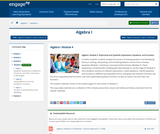Conditional Remix & Share Permitted
CC BY-NC-SA
Rating

In earlier modules, students analyze the process of solving equations and developing fluency in writing, interpreting, and translating between various forms of linear equations (Module 1) and linear and exponential functions (Module 3). These experiences combined with modeling with data (Module 2), set the stage for Module 4. Here students continue to interpret expressions, create equations, rewrite equations and functions in different but equivalent forms, and graph and interpret functions, but this time using polynomial functions, and more specifically quadratic functions, as well as square root and cube root functions.

Subject:
Math
Material Type:
Module
Provider:
New York State Education Department
Provider Set:
EngageNY
09/17/2013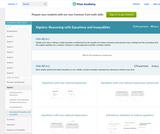Conditional Remix & Share Permitted
CC BY-NC-SA
Rating

This site teaches Reasoning with Equations and Inequalities to High Schoolers through a series of 5909 questions and interactive activities aligned to 36 Common Core mathematics skills.

Subject:
Math
Material Type:
Activity/Lab
Provider:
Provider Set:
10/10/2018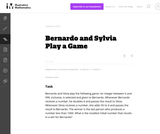Unrestricted Use
CC BY
Rating

This task presents a simple but mathematically interesting game whose solution is a challenging exercise in creating and reasoning with algebraic inequalities. The core of the task involves converting a verbal statement into a mathematical inequality in a context in which the inequality is not obviously presented, and then repeatedly using the inequality to deduce information about the structure of the game.

Subject:
Math
Material Type:
Activity/Lab
Provider:
Illustrative Mathematics
Provider Set:
Illustrative Mathematics
Author:
Illustrative Mathematics
10/21/2013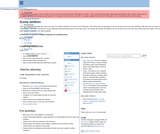Conditional Remix & Share Permitted
CC BY-NC-SA
Rating

This lesson integrates language arts, music, and math. The children will listen to the story "Count on Bunnies". They will be given the opportunity to act out the story and solve bunny equations. After listening to the song "Five Young Rabbits," the children will take turns being rabbits and pantomiming the actions as the class sings. The children will combine the rabbits at the end of each verse to see how many rabbits have been added. Then they will work in pairs to create their own rabbit equations.

Subject:
Arts Education
Education
Math
Social Studies
Material Type:
Lesson
Provider:
University of North Carolina at Chapel Hill School of Education
Provider Set:
LEARN NC Lesson Plans
Author:
Becky Smith
06/25/1999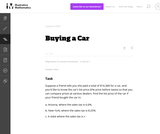Unrestricted Use
CC BY
Rating

The emphasis in this task is on the progression of equations, from two that involve different values of the sales tax, to one that involves the sales tax as a parameter. It is designed to foster the habit of looking for regularity in solution procedures, so that students don't approach every equation as a new problem but learn to notice familiar types.

Subject:
Math
Material Type:
Activity/Lab
Provider:
Illustrative Mathematics
Provider Set:
Illustrative Mathematics
Author:
Illustrative Mathematics
05/01/2012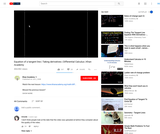Conditional Remix & Share Permitted
CC BY-NC-SA
Rating

This 8-minute video lesson looks at finding the equation of the line tangent to f(x)=xe^x when x=1.

Subject:
Calculus
Material Type:
Lesson
Provider:
Author:
Salman Khan
02/20/2011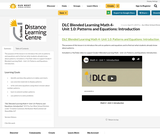Conditional Remix & Share Permitted
CC BY-NC-SA
Rating

The purpose of this lesson is to introduce the unit on patterns and equations and to find out what students already know about patterns.

Included is a YouTube video to support Grade 4 Blended Learning Math - Unit 1.0: Patterns and Equations: Introduction.

Subject:
Math
Material Type:
Activity/Lab
Homework/Assignment
Lesson
Provider:
Sun West Distance Learning Centre (DLC)
Author:
Sun West School Division
04/09/2019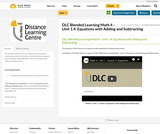Conditional Remix & Share Permitted
CC BY-NC-SA
Rating

The purpose of this lesson is to make and solve equations involving subtraction.

Included is a YouTube video to support Grade 4 Blended Learning Math - Unit 1.4: Equations with Adding and Subtracting.

Subject:
Math
Material Type:
Activity/Lab
Homework/Assignment
Lesson
Provider:
Sun West Distance Learning Centre (DLC)
Author:
Sun West School Division
04/09/2019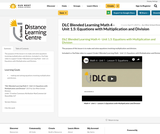Conditional Remix & Share Permitted
CC BY-NC-SA
Rating

The purpose of this lesson is to make and solve equations involving multiplication and division.

Included is a YouTube video to support Grade 4 Blended Learning Math - Unit 1.5: Equations with Multiplication and Division.

Subject:
Math
Material Type:
Activity/Lab
Homework/Assignment
Lesson
Provider:
Sun West Distance Learning Centre (DLC)
Author:
Sun West School Division
04/09/2019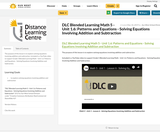Conditional Remix & Share Permitted
CC BY-NC-SA
Rating

The purpose of this lesson is to explore solving equations involving addition and subtraction.

Included is a YouTube video to support Grade 5 Blended Learning Math - Unit 1.6: Patterns and Equations - Solving Equations Involving Addition and Subtraction.

Subject:
Math
Material Type:
Activity/Lab
Homework/Assignment
Lesson
Provider:
Sun West Distance Learning Centre (DLC)
Author:
Sun West School Division
05/10/2019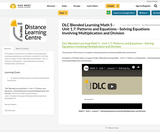Conditional Remix & Share Permitted
CC BY-NC-SA
Rating

The purpose of this lesson is to explore solving equations involving multiplication and division.

Included is a YouTube video to support Grade 5 Blended Learning Math - Unit 1.7: Patterns and Equations - Solving Equations Involving Multiplication and Division.

Subject:
Math
Material Type:
Activity/Lab
Homework/Assignment
Lesson
Provider:
Sun West Distance Learning Centre (DLC)
Author:
Sun West School Division
05/10/2019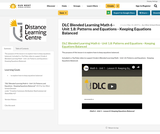Conditional Remix & Share Permitted
CC BY-NC-SA
Rating

The purpose of this lesson is to explore how to keep equations balanced.

Included is a YouTube video to support Grade 6 Blended Learning Math - Unit 1.8: Patterns and Equations - Keeping Equations Balanced.

Subject:
Math
Material Type:
Activity/Lab
Homework/Assignment
Lesson
Provider:
Sun West Distance Learning Centre (DLC)
Author:
Sun West School Division
05/14/2019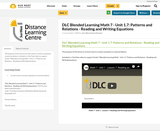Conditional Remix & Share Permitted
CC BY-NC-SA
Rating

The purpose of this lesson is to learn how to connect equations to word problems.

Included is a YouTube video to support Grade 7 Blended Learning Math - Unit 1.7: Patterns and Relations - Reading and Writing Equations.

Subject:
Math
Material Type:
Activity/Lab
Homework/Assignment
Lesson
Provider:
Sun West Distance Learning Centre (DLC)
Author:
Sun West School Division
06/07/2019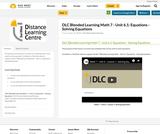Conditional Remix & Share Permitted
CC BY-NC-SA
Rating

The purpose of this lesson is to learn two methods that can be used to solve equations.

Included is a YouTube video to support Grade 7 Blended Learning Math - Unit 6.1: Equations - Solving Equations.

Subject:
Math
Material Type:
Activity/Lab
Homework/Assignment
Lesson
Provider:
Sun West Distance Learning Centre (DLC)
Author:
Sun West School Division